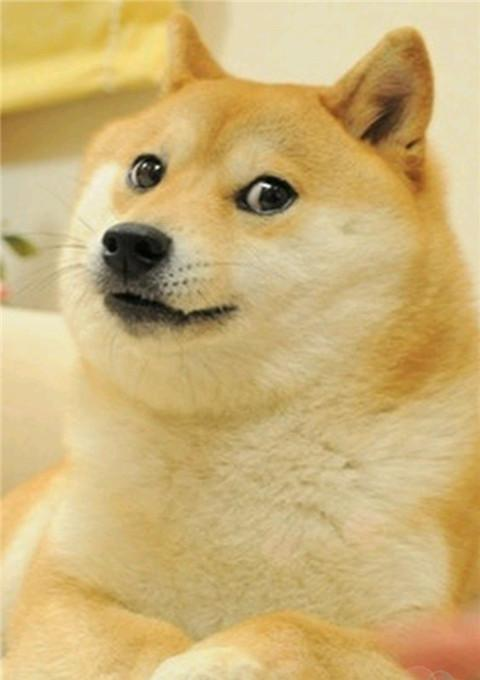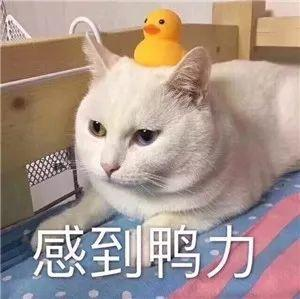## 0x02 相对熵（KL散度）

### KL散度计算问题

• 当 $p_i = 0$ 时。$\lim_{p\to 0}{p\log{p}} = 0$
• 当 $p_i \not ={0}$ 但是 $q_i = 0$ 时。这个时候认为 $D_{KL}(p|q)=\infty$

$p_i$ 或 $q_i$ 取0的问题，在用代码计算上不至于太难解决，只要保证每一项都能加上一个足够小的非零正数即可。

## 0x04 例子category dog cat duck
Label 1 0 0
Predicts 0.7 0.2 0.1category dog cat duck
Label 0 1 1
Predicts 0.2 0.8 0.5